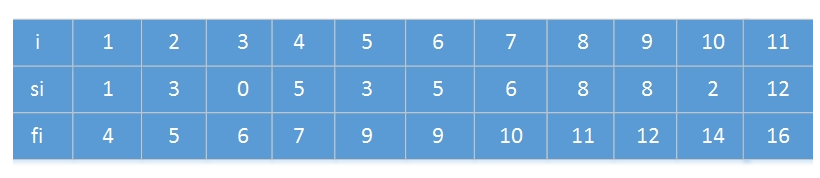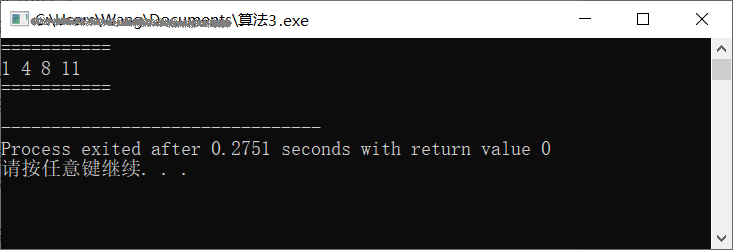IT数码 购物 网址 头条 软件 日历 阅读 图书馆
 TxT小说阅读器 ↓语音阅读,小说下载,古典文学↓ 图片批量下载器 ↓批量下载图片,美女图库↓ 图片自动播放器 ↓图片自动播放器↓ 一键清除垃圾 ↓轻轻一点,清除系统垃圾↓
 开发: C++知识库 Java知识库 JavaScript Python PHP知识库 人工智能 区块链 大数据 移动开发 嵌入式 开发工具 数据结构与算法 开发测试 游戏开发 网络协议 系统运维 教程: HTML教程 CSS教程 JavaScript教程 Go语言教程 JQuery教程 VUE教程 VUE3教程 Bootstrap教程 SQL数据库教程 C语言教程 C++教程 Java教程 Python教程 Python3教程 C#教程 数码: 电脑 笔记本 显卡 显示器 固态硬盘 硬盘 耳机 手机 iphone vivo oppo 小米 华为 单反 装机 图拉丁
 -> 数据结构与算法 -> 算法基础之贪心 -> 正文阅读

### [数据结构与算法]算法基础之贪心

 贪心算法（greedy algorithm），是用计算机来模拟一个“贪心”的人做出决策的过程。这个人十分贪婪，每一步行动总是按某种指标选取最优的操作。 贪心算法，又名贪婪法，是寻找最优解问题的常用方法，这种方法模式一般将求解过程分成若干个步骤，但每个步骤都应用贪心原则，选取当前状态下最好/最优的选择（局部最有利的选择），并以此希望最后堆叠出的结果也是最好/最优的解。 贪心算法的基本思路： ??? 1.建立数学模型来描述问题。 ??? 2.把求解的问题分成若干个子问题。 ??? 3.对每一子问题求解，得到子问题的局部最优解。 4.把子问题的解局部最优解合成原来解问题的一个解。 示例1 老魏开了间小店，不能电子支付，钱柜里的货币只有 25 分、10 分、5 分和 1 分四种硬币，如果你是售货员且要找给客户42分钱的硬币，如何安排才能找给客人的钱既正确且硬币的个数又最少？ 解析： （1）建立数学模型 选择硬币面额的和= 42. （2）问题拆分为子问题 选择硬币进行支付的过程，可以划分为n个子问题：即每个子问题对应为： 在未超过51的前提下，在剩余的硬币中选择一张硬币。 （3）制定贪心策略，求解子问题 初次先找给顾客25分，还差顾客sum_money=42-25=17，不能再选25分的； 选10分的，此时sum_money=17-10=7，还能够选10分的； 选5分的，此时sum_money=7-5=2，不能再选5分的； 选1分的，此时sum_money=2-1=1。还能够选10分的； sum_money=1-1=0至此，顾客收到零钱，交易结束。 （4）将所有解元素合并为原问题的解 此时42分，分成了1个25，2个10，1个5，1个2，共四枚硬币。 源码 ``````#include using namespace std; #define ONEFEN 1 #define FIVEFEN 5 #define TENFEN 10 #define TWENTYFINEFEN 25 int main() { int sum_money=42; int num_25=0,num_10=0,num_5=0,num_1=0; //不断尝试每一种硬币 while(sum_money>=TWENTYFINEFEN) { num_25++; sum_money -=TWENTYFINEFEN; } while(sum_money>=TENFEN) { num_10++; sum_money -=TENFEN; } while(sum_money>=FIVEFEN) { num_5++; sum_money -=FIVEFEN; } while(sum_money>=ONEFEN) { num_1++; sum_money -=ONEFEN; } //输出结果 cout<< "25分硬币数："<=fj或sj>=fi，则ai和aj是兼容的。在活动选择问题中，我们希望选出一个最大兼容活动集。假定活动已按结束时间fi的单调递增顺序排序： ?? f1<=f2<=f3<=f4<=...<=fn-1<=fn 考虑下面的活动集合：我们可以给出贪心算法在解决这个问题的两种方式：递归和迭代方式，两种算法都是按照自顶向下来求解问题的。 源代码如下： ``````#include #include using namespace std; void swap(int* a, int* b) { int tmp = *a; *a = *b; *b = tmp; } int Adjust_Arr(int* a, int* b, int start, int end) { int p = start; int q = end; int i = p - 1; int j = p; int key = a[q]; while (j < q) { if (a[j] >= key) { j++; continue; } else { i++; swap(a + i, a + j); swap(b + i, b + j); j++; } } i++; swap(a + i, a + q); swap(b + i, b + q); return i; } void Quick_Sort(int* a, int* b, int start, int end) { if (start < end) { int mid = Adjust_Arr(a, b, start, end); Quick_Sort(a, b, start, mid - 1); Quick_Sort(a, b, mid + 1, end); } } void Print_Arr(int* a, int len) { for (int i = 0; i < len; i++) { cout << a[i] << ' '; } cout << endl; } // 递归版本 void Recursive_Activity_Selector(vector* A, int* s, int* f, int k, int n) { int m = k + 1; while (m <= n && s[m] < f[k]) { m++; } if (m <= n) { A->push_back(m); Recursive_Activity_Selector(A, s, f, m, n); } } // 迭代版本 vector* Greedy_Activity_Selector(int*s, int*f, int n) { vector* A = new vector; int k = 1; A->push_back(k); for (int m = 2; m <= n; m++) { if (s[m] >= f[k]) { A->push_back(m); k = m; } } return A; } int main() { int s = { 0, 1, 3, 0, 5, 3, 5, 6, 8, 8, 2, 12 }; int f = { 0, 4, 5, 6, 7, 9, 9, 10, 11, 12, 14, 16 }; //先将f按从小到大排序，s的位置随f而动 Quick_Sort(f, s, 0, 12 - 1); //下面两句用作调用递归版本 // vector* A = new vector; // Recursive_Activity_Selector(A, s, f, 0, 12 - 1); //这里下标只能取到11 //下面一句用作调用迭代版本 vector* A = Greedy_Activity_Selector(s, f, 12 - 1); cout << "===========" << endl; vector::iterator iter; for (iter = A->begin(); iter != A->end(); iter++) { cout << *iter << ' '; } cout << endl << "===========" << endl; delete A; return 0; } `````` 运行之，结果如下：由此可知，本例中，{a1,a4,a8,a11}是一个最大集。
 上一篇文章      下一篇文章      查看所有文章
 加:2021-08-20 15:22:16  更:2021-08-20 15:22:21
 开发: C++知识库 Java知识库 JavaScript Python PHP知识库 人工智能 区块链 大数据 移动开发 嵌入式 开发工具 数据结构与算法 开发测试 游戏开发 网络协议 系统运维 教程: HTML教程 CSS教程 JavaScript教程 Go语言教程 JQuery教程 VUE教程 VUE3教程 Bootstrap教程 SQL数据库教程 C语言教程 C++教程 Java教程 Python教程 Python3教程 C#教程 数码: 电脑 笔记本 显卡 显示器 固态硬盘 硬盘 耳机 手机 iphone vivo oppo 小米 华为 单反 装机 图拉丁
 360图书馆 购物 三丰科技 阅读网 日历 万年历 2021年10日历 -2021/10/28 13:48:18-
 图片自动播放器 ↓图片自动播放器↓ TxT小说阅读器 ↓语音阅读,小说下载,古典文学↓ 一键清除垃圾 ↓轻轻一点,清除系统垃圾↓ 图片批量下载器 ↓批量下载图片,美女图库↓
 网站联系: qq:121756557 email:121756557@qq.com  IT数码